# Circular Trigonometry Function Lesson Plan | Class 11 Math Lesson Plan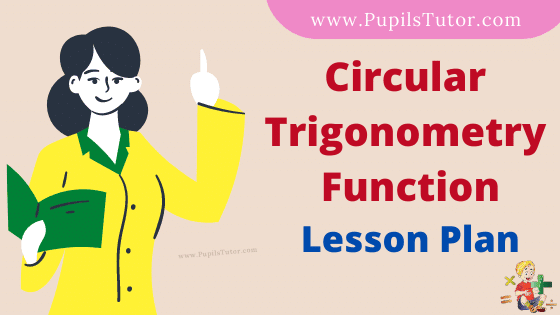Hello Friends, Welcome To Our Website. How Are You? Hope You Are Doing Well,

If You Are Searching For Circular Trigonometry Function Lesson Plan Then You Have Come To The Right Place. Here We Have Shared The Lesson Plan On Circular Trigonometry Function For B.Ed, D.El.Ed, And School Teachers.

This Real School Teaching Mathematics Lesson Plan On Circular Trigonometry Functions Is Specially Made For The B.Ed 1st And 2nd Year Students But All The School Teachers And Trainee Teacher Of All Classes Can Prepare Their Final Teaching Plan Very Easily With The Help Of This Sample Circular Trigonometry Function Lesson Plan For Class 11th And 12th.

Brief Overview Of The Lesson Plan

 Class 11 Topic Circular Trigonometry Function Subject Maths Lesson Plan Type Real School Teaching Skill Real School Teaching And Practice

The Topics And Points That Are Covered In This Mathematic Lesson Plan On Circular Trigonometry Function Are : Trigonometric Functions, Circular Functions, Quadrants, First Quadrant, Second Quadrant, Third Quadrant, Fourth Quadrant with Suitable Examples And Question Answers…..

## Circular Trigonometry Function Lesson Plan –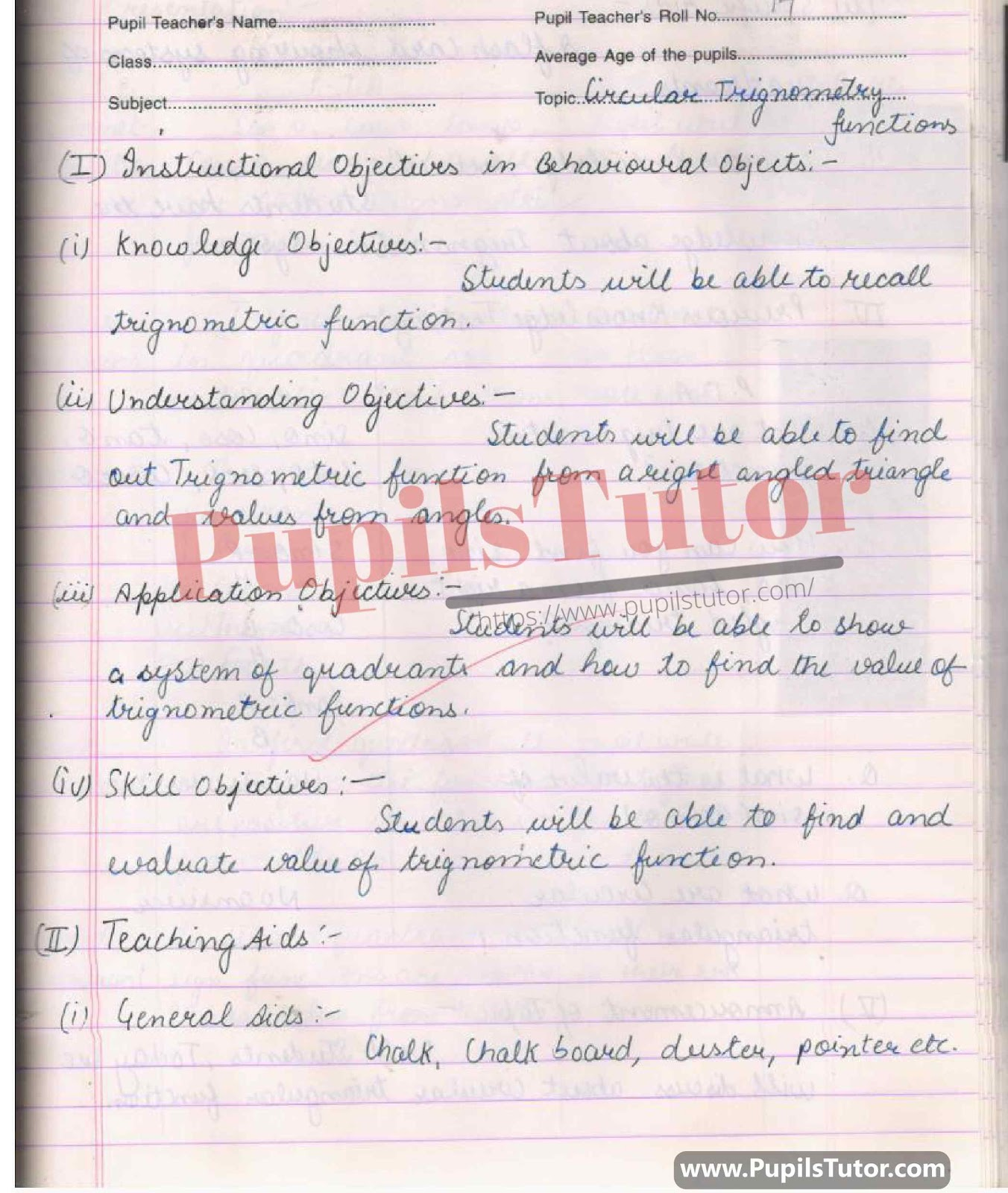## Mega And Real School Teaching Mathematics Lesson plan On Circular Trigonometry Function, Trigonometry Quadrants For All B.Ed And Deled Students In English Free Download PDF And PPT (PowerPoint Presentation And Slides) –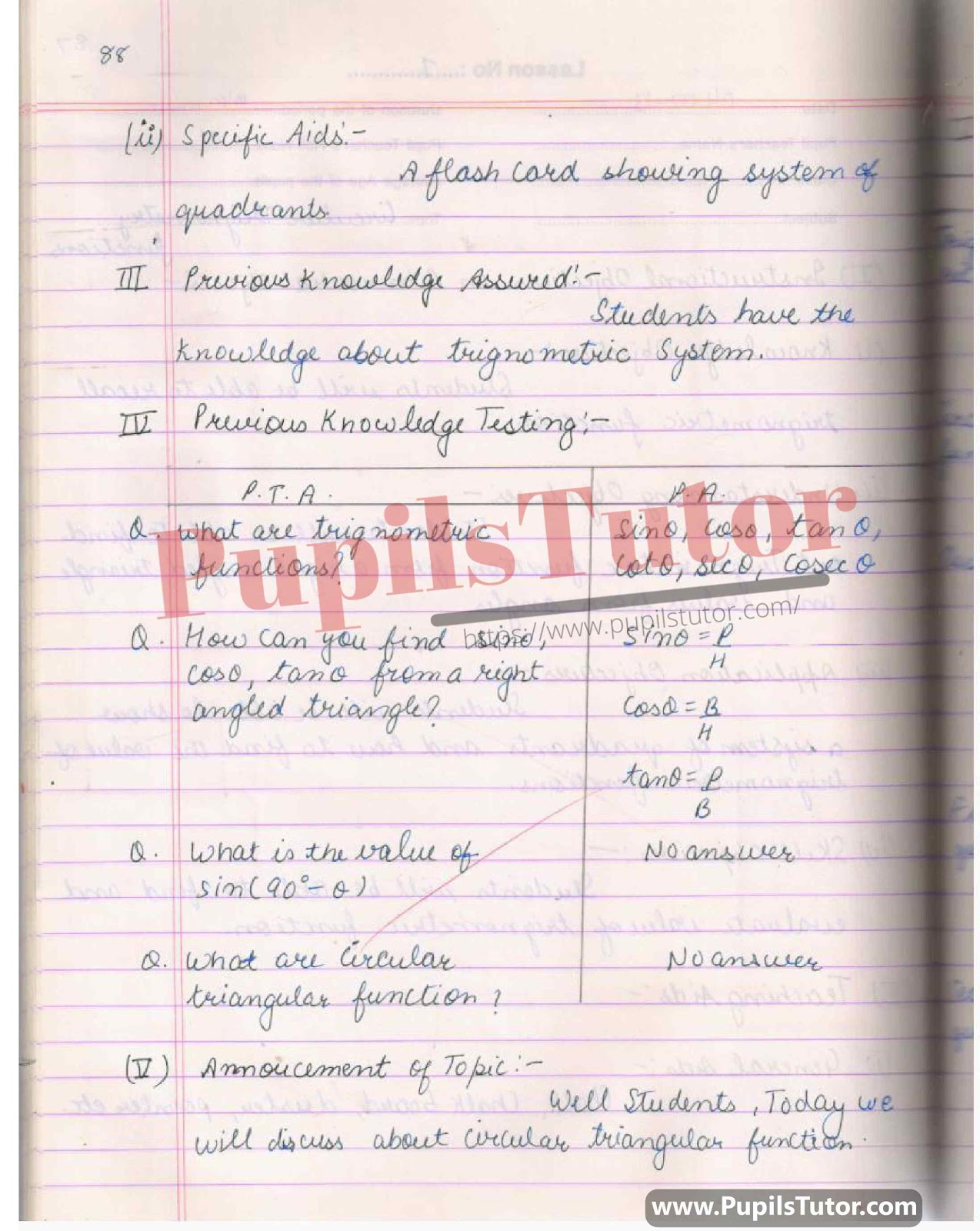## Math Lesson Plan On Sin, Cos, Tan, Cot, Cosec, Sec, Trigno Angle, Circular Trigno Function For Class/Grade 11, 12 For CBSE NCERT School And College Teachers –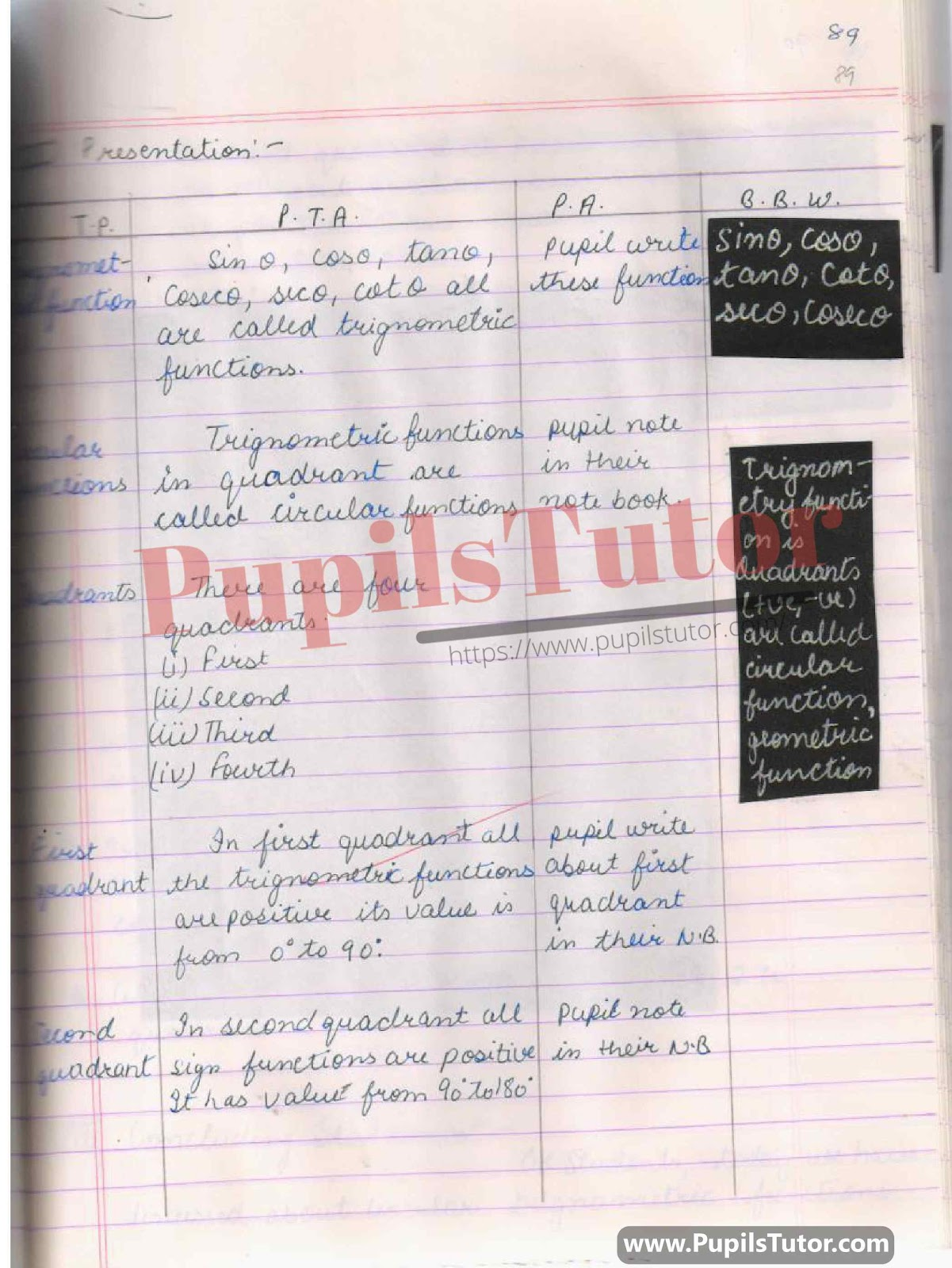## BED, DELED, BTC, BSTC, M.ED, DED And NIOS Teaching Of Math Innovative Digital Lesson Plan Format On Trigno Function, Circular Trigno Functions, Quadrant, 1st Quadrant, 2nd Quadrant, 3rd Quadrant, 4th Quadrant Topic For Class 9th, 10th, 11th, 12th –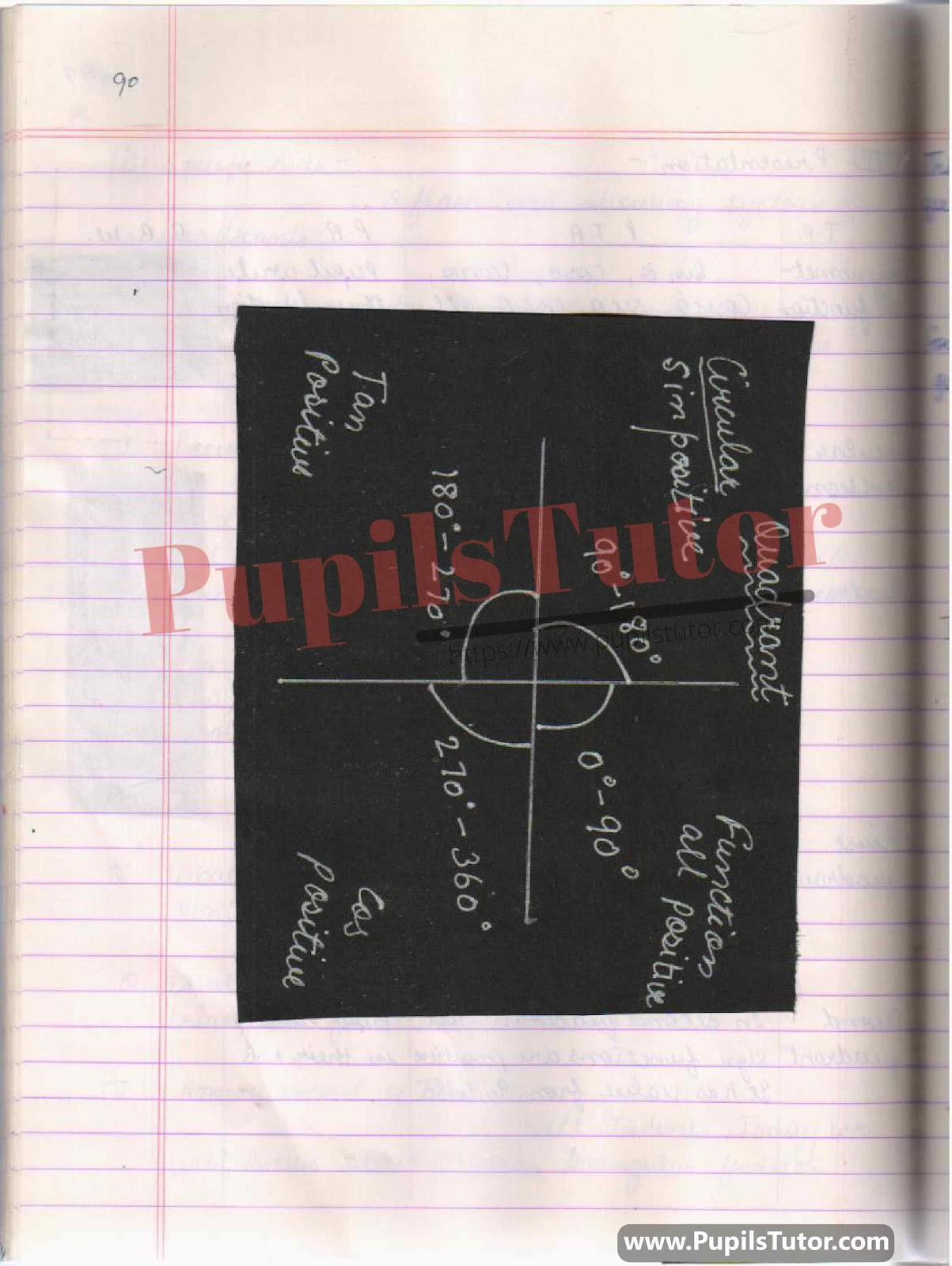## Lesson Plan On Circular Trigonometry Function For Class 11th. –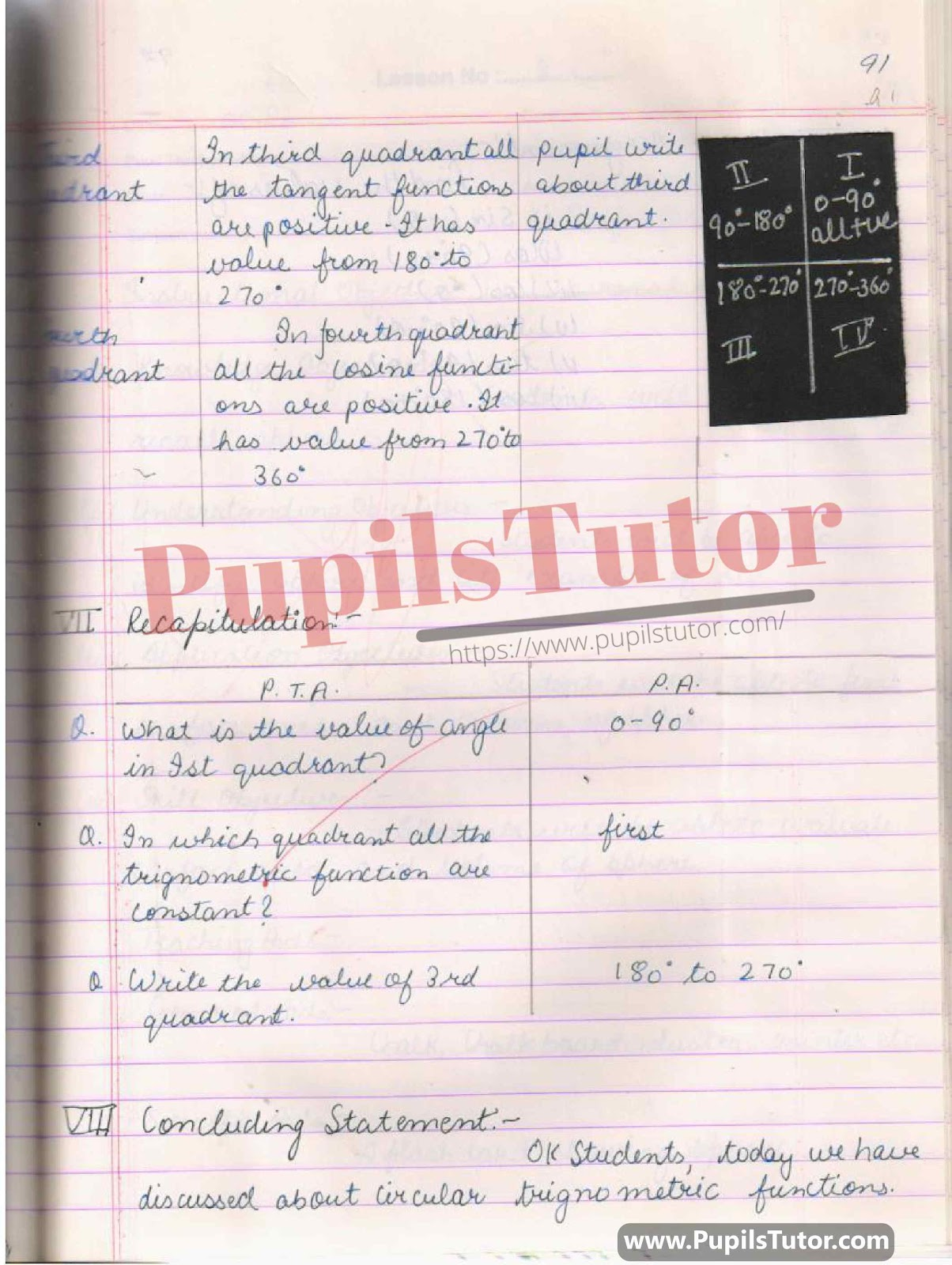## Circular Trigonometry Function Lesson Plan For B.Ed 1st Year, 2nd Year And All Semesters Students –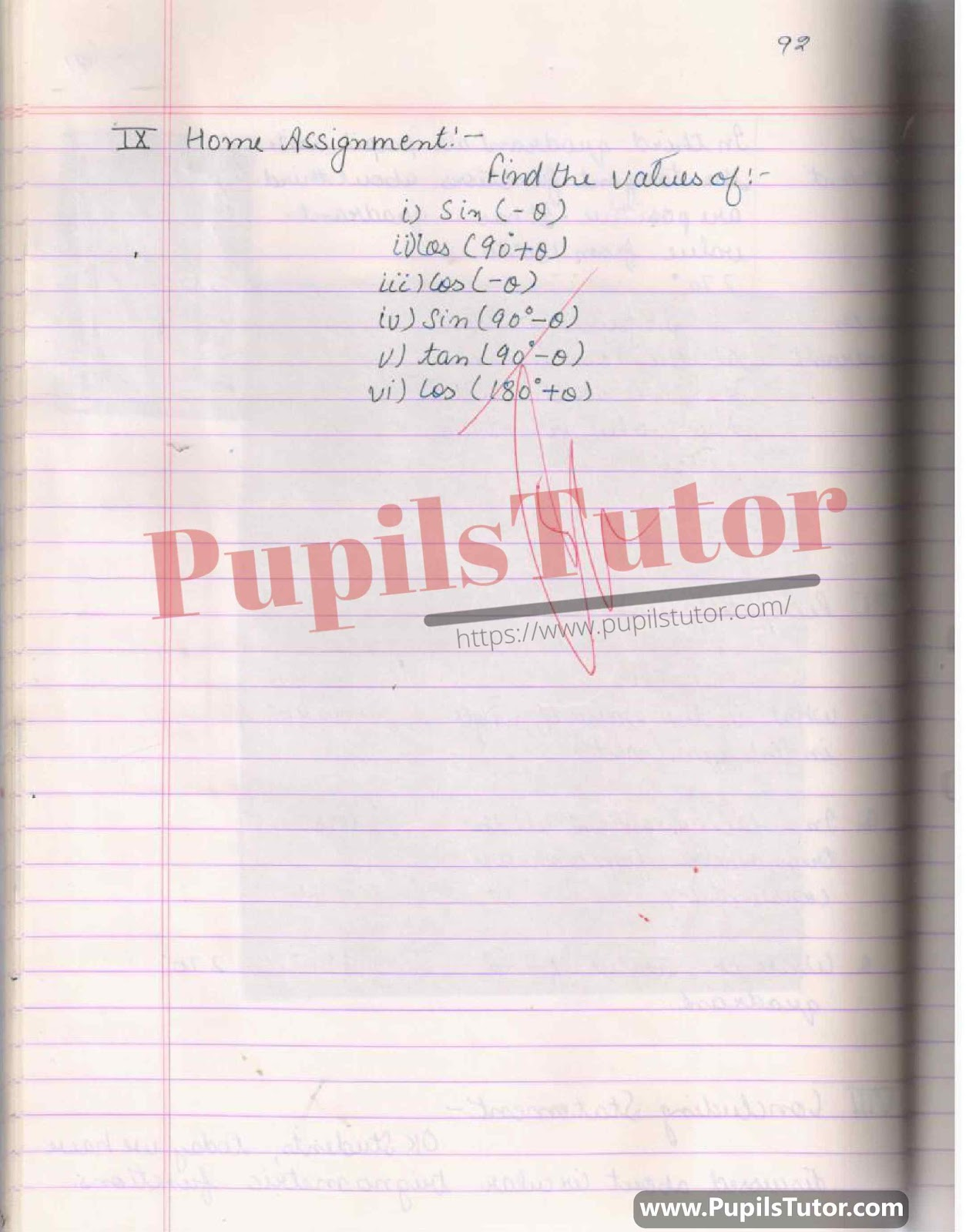You Might Also Like:

Further Reference

 B.Ed. Question Bank And Exam Notes A Complete Guide For B.ED. Entrance Examination CTET & TETs Preparation Material Teaching Aptitude and Attitude Test Book UP B.Ed Guidebook & Latest Practice Sets Book For Entrance Exam B.Ed CET Delhi University B.Ed Entrance Test Latest Practice Sets IGNOU B.Ed. Entrance Test: Previous Years Papers (Solved) Assignments And Files Lesson Planning Files And Guide

On Our Website www.PupilsTutor.Com, We Have Also Shared Lots Of Other Lesson Plans Of All Subjects On Various Teaching Skills Like Micro-Teaching, Mega Teaching, Discussion Skill, Real School Teaching And Practice Skill, Simulated And Observation Skills. You Can Also Check Them.

If You Liked This Circular Trigonometry Function Lesson Plan For Class 11 Then Please Share Our Efforts With Your Friends Also.

You Can Also Share Your Lesson Plans, Assignments, Files, Papers And Study Notes With Us To Help Other Students .

#### List Of Some More Mathematics Lesson Plans

 Profit And Loss B.Ed 2nd Year Lesson Plan For Mathematics Sum Of The Angles Of A Triangle B.Ed 2nd Year Lesson Plan For Math Trigonometric Ratio B.Ed 2nd Year Lesson Plan For Mathematics Circle B.Ed 2nd Year Lesson Plan For Mathematics Properties Of Angle In Circle B.Ed 2nd Year Lesson Plan For Mathematics Pie Chart B.Ed 2nd Year Lesson Plan For Mathematics Three Dimensional Shape B.Ed 2nd Year Lesson Plan For Mathematics Trigonometry B.Ed 2nd Year Lesson Plan For Mathematics Parts Of A Circle B.Ed 2nd Year Lesson Plan For Maths Compound Interest B Ed 2nd Year Lesson Plan For Mathematics Volume Of Cone And Cylinder B.Ed 2nd Year Lesson Plan For Mathematics Areas Related To Circle B.Ed 2nd Year Lesson Plan For Mathematics Integer DELED 2nd Year Lesson Plan For Mathematics Surface Area Of Cone And Cylinder B.Ed 2nd Sem Lesson Plan For Mathematics Statistics And Arithmetic Mean B.Ed 2nd Year Lesson Plan For Mathematics Volume Of Right Circular Cylinder B.Ed 2nd Year Lesson Plan For Mathematics Circular Trigonometry Function B.Ed 2nd Year Lesson Plan For Mathematics 3D Shapes B.Ed 2nd Year Lesson Plan For Mathematics Coordinate Geometry Section Formula B.Ed 2nd Year Lesson Plan For Mathematics Surface Area And Volume Of Sphere B.Ed 2nd Year Lesson Plan For Mathematics Theorem M.Ed 1st Year Lesson Plan For Mathematics Curved And Total Surface Area Of Cylinder BEd 2nd Year Lesson Plan For Mathematics Quadrilateral B.Ed 2nd Year Lesson Plan For Math Height And Distance B.Ed 2nd Year Lesson Plan For Mathematics Curved And Total Surface Area Of Cone B.Ed 2nd Year Lesson Plan For Mathematics Coordinate Geometry B.Ed 2nd Year Lesson Plan For Mathematics Similar Triangle BEd 2nd Year Lesson Plan For Mathematics Polynomials B.Ed 2nd Year Lesson Plan For Mathematics Trigonometry Transformation Formula B.Ed First Year Lesson Plan For Mathematics Surface Area Of Cube And Cuboid B.Ed 2nd Semester Lesson Plan For Mathematics Algebraic Expression B.Ed Second Year Lesson Plan For Mathematics Proportion B.Ed 2nd Year Lesson Plan For Mathematics